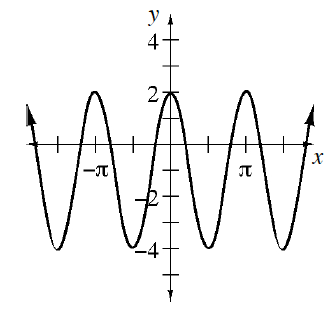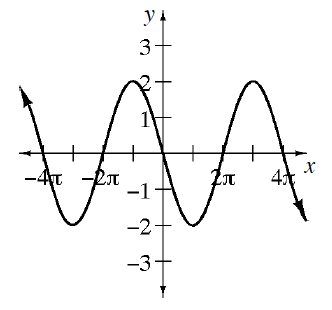### Home > PC3 > Chapter 7 > Lesson 7.2.1 > Problem7-79

7-79.

Write an equation for each graph below.

1.This looks like a cosine curve with a period of $π$ that has been stretched and shifted vertically.

To determine the vertical shift, sketch the midline.
The amplitude can be determined by figuring out how far above the midline the maximum points are located.

1.This looks like a sine curve with a period of $4\pi$, an amplitude of $2$, and is vertically reflected.